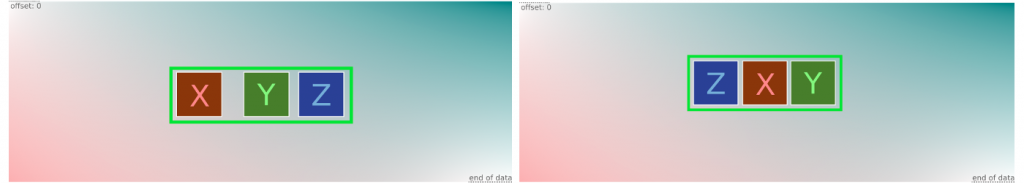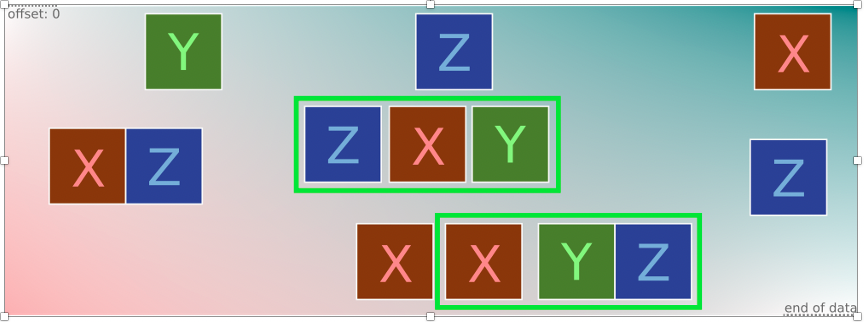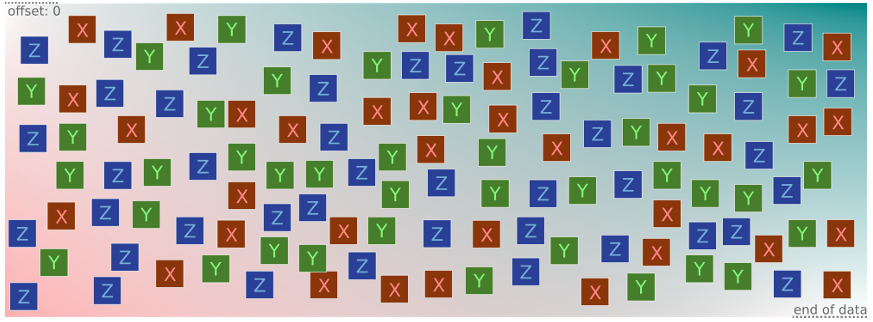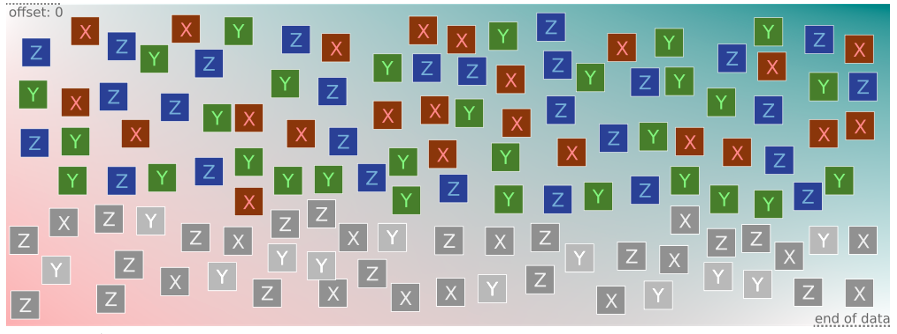# Threat hunting with Yara: Measuring distance between any three elements

By on 16 Mar 2022

Category: Tech mattersThis series explores real-life examples of advanced Yara uses, seeking to generalize them to form an abstract problem. It also explores general approaches to solve such uses while examining the pros and cons of possible solutions. The material provided here should be educational for those new to Yara but should also be suitable for very experienced Yara users as it uncovers some fundamental issues.

In my first post, we looked at a couple of real-life research problems — detecting code evolution and shellcodes — before generalizing the problem definition.

Now, let’s assume that any pattern can be the first or the last by limiting the distance between the three. To do so, we will use Yara’s math module and min/max functions from it:

`import "math"rule three_body_problem_3 {  meta:    description = "Rule to detect 3 patterns in arbitrary order, while limiting the distance between them."strings:  \$x = { 11 11 11 11 }  \$y = { 22 22 22 22 }  \$z = { 33 33 33 33 }condition:  all of them andmath.max(math.max(@x,@y),@z) - math.min(math.min(@x,@y),@z) <= 16}`

### Pros:

• The rule detects patterns X, Y, Z in arbitrary order while limiting the distance between the start and the end of the X, Y, Z sequence (again, in arbitrary order).Figure 1 — Detected X, Y, Z that are not too far from each other and in any order.

### Cons:

• This rule misses some files. The problem appears when one of the patterns is located apart from the target sequence. It becomes the first or the last and breaks the detection.

## Iterating through all the matches

The previous rule fails to detect cases when a file contains more than one of the target patterns (Figure 1) because it only checks the first matched pattern Z, ignoring the second Z and potential others.

Given that we cannot predict how many matches of the same pattern we have after the scan, we should check them all. Luckily for us, Yara puts all matches in an array that can be accessed in the condition section using an iterator. If you are new to Yara iterators, take a moment to read about this with examples in the official documentation.

There is also a special prefix # in Yara that allows access to the count of matches per pattern. Let’s check the following refined rule:

`import "math"rule three_body_problem_4 {  meta:    description = "Rule to detect 3 patterns in arbitrary order, while limiting the distance between them. It checks the distance between all the matches and their combinations."strings:  \$x = { 11 11 11 11 }  \$y = { 22 22 22 22 }  \$z = { 33 33 33 33 }condition:  all of them and   for any i in (1..#x):  (    for any j in (1..#y):    (      for any k in (1..#z):      (        math.max(math.max(@x[i],@y[j]),@z[k]) -math.min(math.min(@x[i],@y[j]),@z[k]) <= 16        )      )    )}`

### Pros:

• The rule detects all X, Y, Z patterns in any order while limiting the distance between the start and the end of the X, Y, Z sequence.Figure 3 — Detected X, Y, Z patterns that are not too far from each other and in any order.

### Cons:

• Unfortunately, this rule has serious performance issues if there are many matches per pattern. Condition checks, including iterations in Yara, are implemented through generating and executing an internal virtual machine (VM) code. While the VM brings a lot of opportunities in terms of Yara language features, it can become a significant performance killer when you need to process a large number of elements even for a small file.

Given that we have #x, #y, and #z count of matches for each X, Y, Z pattern, respectively, the overall number of comparison iterations will be #x*#y*#z. This would result in one billion comparisons for 1000 matches of each of the three patterns per file.

Considering that the VM runs only in a single thread and caches the results of some function calls, such a rule, with a poor choice of patterns or specially crafted files, can ruin your scanning process by taking an enormously long time or even crash your system due to lack of memory.

We ran a test with 1,000 matches per each X, Y, Z pattern in a small file (12 KB file) and the scanner ran for ~10 minutes before being forcibly killed by the OS due to overuse of the RAM. The scanner silently exited due to the exception.Figure 4 — Too many pattern matches kill the scanning process.

## Iterations count threshold

We could prevent hitting the bottleneck of the previous rule by limiting the number of matches to a maximum threshold per pattern as follows:

`import "math"rule three_body_problem_5 {  meta:    description = "Rule to detect 3 patterns in arbitrary order, while limiting the distance between them. It checks the distance between all the matches and their combinations within a reasonable threshold."strings:  \$x = { 11 11 11 11 }  \$y = { 22 22 22 22 }  \$z = { 33 33 33 33 }condition:  all of them and   for any i in (1..math.min(#x,100)):  (    for any j in (1..math.min(#y,100)):    (      for any k in (1..math.min(#z,100)):      (        math.max(math.max(@x[i],@y[j]),@z[k]) -math.min(math.min(@x[i],@y[j]),@z[k]) <= 16        )      )    )}`

### Pros:

• This rule doesn’t hang even if the scanner comes across too many matches.

### Cons:

• We are closing our eyes on some border cases and may miss detections of certain files.
• Hard limits for every pattern set to 100 doesn’t mean that we are limited by hundreds of thousands of max iterations. Take, for example, #x=101, #y=1, #z=1. The product is 101, which is an acceptable number of iterations, but we will check only 100, missing the last one, which might be crucial for the detection.

## Enhanced iterations count threshold

If we keep chasing this approach, we can improve the overall threshold usage and also report every file that crossed the maximum threshold. Such files can be dealt with separately, perhaps, without running an unbearable number of comparisons in the Yara VM. Here is how you do this in Yara:

`import "math"rule three_body_problem_6 {  meta:    description = "Rule to detect 3 patterns in arbitrary order, while limiting the distance between them. It checks the distance between all the matches and their combinations. An enhanced iterations threshold is preset."strings:  \$x = { 11 11 11 11 }  \$y = { 22 22 22 22 }  \$z = { 33 33 33 33 }condition:  all of them and#x*#y*#z >= 1000000 or   for any i in (1..#x):  (    for any j in (1..#y):    (      for any k in (1..#z):      (        math.max(math.max(@x[i],@y[j]),@z[k]) -math.min(math.min(@x[i],@y[j]),@z[k]) <= 16        )      )    )}`

### Pros:

• This rule doesn’t hang even if the scanner comes across too many matches.

### Cons:

• If there are more than 100 of each pattern matches, which is possible when scanning a memory dump or a raw disk image, this doesn’t bring much value because the rule will be triggered due to the threshold being reached. The process also requires some additional external validation of the data.Figure 5 — The rule processes only part of the matches.

In my next post, I will look at dealing with wildcard hexadecimal patterns.

## Appendix: Yara test set file generator

Should you like to try out the proposed solution or keep finding the best one, feel free to use the following python script that generates initial test files with some special cases mentioned in this series. Note, that good solutions must detect all sample_good* files and no sample_bad* files.

```X = b"\x11\x11\x11\x11"
Y = b"\x22\x22\x22\x22"
Z = b"\x33\x33\x33\x33"
J = b" " #Junk or blank space

with open("sample_good1.dat","wb") as f:
f.write( X + Y + Z ) and f.close()

with open("sample_good2.dat","wb") as f:

f.write( Y + X + J*100 + X + Y + Z ) and f.close()

with open("sample_good3.dat","wb") as f:
f.write( Z + J*4 + Y + X ) and f.close()

with open("sample_good4.dat","wb") as f:
f.write( (X+J)*90+ J*20 + X + J*4 + Y + J*4 + Z ) and f.close()

with open("sample_good5.dat","wb") as f:
f.write( X + J*4 + Y + J*4 + Z + \
J*100 + X + J*4 + Y + Z + \
J*100 + X + Y + Z )
f.close()

with open("sample_good6.dat","wb") as f:
f.write( (X+J)*100 + J*2 + Y + J*4 + Z ) and f.close()

with open("sample_bad1.dat","wb") as f:
f.write( X + 16*J + Y + 16*J + Z ) and f.close()

with open("sample_bad2.dat","wb") as f:
f.write( X + 8*J + Y ) and f.close()

with open("sample_bad3.dat","wb") as f:
f.write( X + 8*J + Y + 8*J + Z ) and f.close()

with open("sample_bad4.dat","wb") as f:
f.write( ( X + 8*J + Y + 8*J + Z+8*J) * 1000 ) and f.close()

with open("sample_bad5.dat","wb") as f:
f.write( ( X*100 + 8*J + Y + 8*J + Z*100) * 1278) and f.close()```

Vitaly Kamluk is Director of the Global Research and Analysis Team for Kaspersky Asia Pacific.